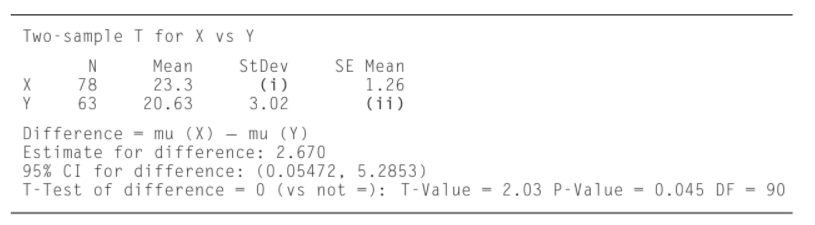×
Get Full Access to Statistics For Engineers And Scientists - 4 Edition - Chapter 6.5 - Problem 13e
Get Full Access to Statistics For Engineers And Scientists - 4 Edition - Chapter 6.5 - Problem 13e

×

# The following MINITAB output presents the | Ch 6.5 - 13EISBN: 9780073401331 38

## Solution for problem 13E Chapter 6.5

Statistics for Engineers and Scientists | 4th Edition

• Textbook Solutions
• 2901 Step-by-step solutions solved by professors and subject experts
• Get 24/7 help from StudySoup virtual teaching assistantsStatistics for Engineers and Scientists | 4th Edition

4 5 1 319 Reviews
26
1
Problem 13E

The following MINITAB output presents the results of a hypothesis test for the difference µx - µy between two population means. Some of the numbers are missing.a. Fill in the missing numbers for (i) and (ii).

b. The output presents a Student’s t test. Compute the P-value using a z test. Are the two results similar?

c. Use the output and an appropriate table to compute a 98% confidence interval for  µx - µy based on the z statistic.

Step-by-Step Solution:
Step 1 of 3

Psych Measurement Tests of Intelligence Chapter 10 ~Some considerations which may make a test more appealing to a potential administrator of the test: ­ theory on which the test was based ­ how easily the test can be administered ­ how easily the test can be scored ­ how easily test results can be interpreted ­ the test’s utility ­ adequacy/appropriateness of the test norms ­ Reliability and validity indices ~Characteristics of the Stanford Binet Tests (there are a total of V variations of the test): ­ First tests lacked proper representation of the population in the samp

Step 2 of 3

Step 3 of 3

##### ISBN: 9780073401331

This full solution covers the following key subjects: test, output, results, compute, presents. This expansive textbook survival guide covers 153 chapters, and 2440 solutions. This textbook survival guide was created for the textbook: Statistics for Engineers and Scientists , edition: 4. Statistics for Engineers and Scientists was written by and is associated to the ISBN: 9780073401331. The full step-by-step solution to problem: 13E from chapter: 6.5 was answered by , our top Statistics solution expert on 06/28/17, 11:15AM. The answer to “?The following MINITAB output presents the results of a hypothesis test for the difference µx - µy between two population means. Some of the numbers are missing. a. Fill in the missing numbers for (i) and (ii).b. The output presents a Student’s t test. Compute the P-value using a z test. Are the two results similar?c. Use the output and an appropriate table to compute a 98% confidence interval for µx - µy based on the z statistic.” is broken down into a number of easy to follow steps, and 78 words. Since the solution to 13E from 6.5 chapter was answered, more than 336 students have viewed the full step-by-step answer.

Unlock Textbook Solution